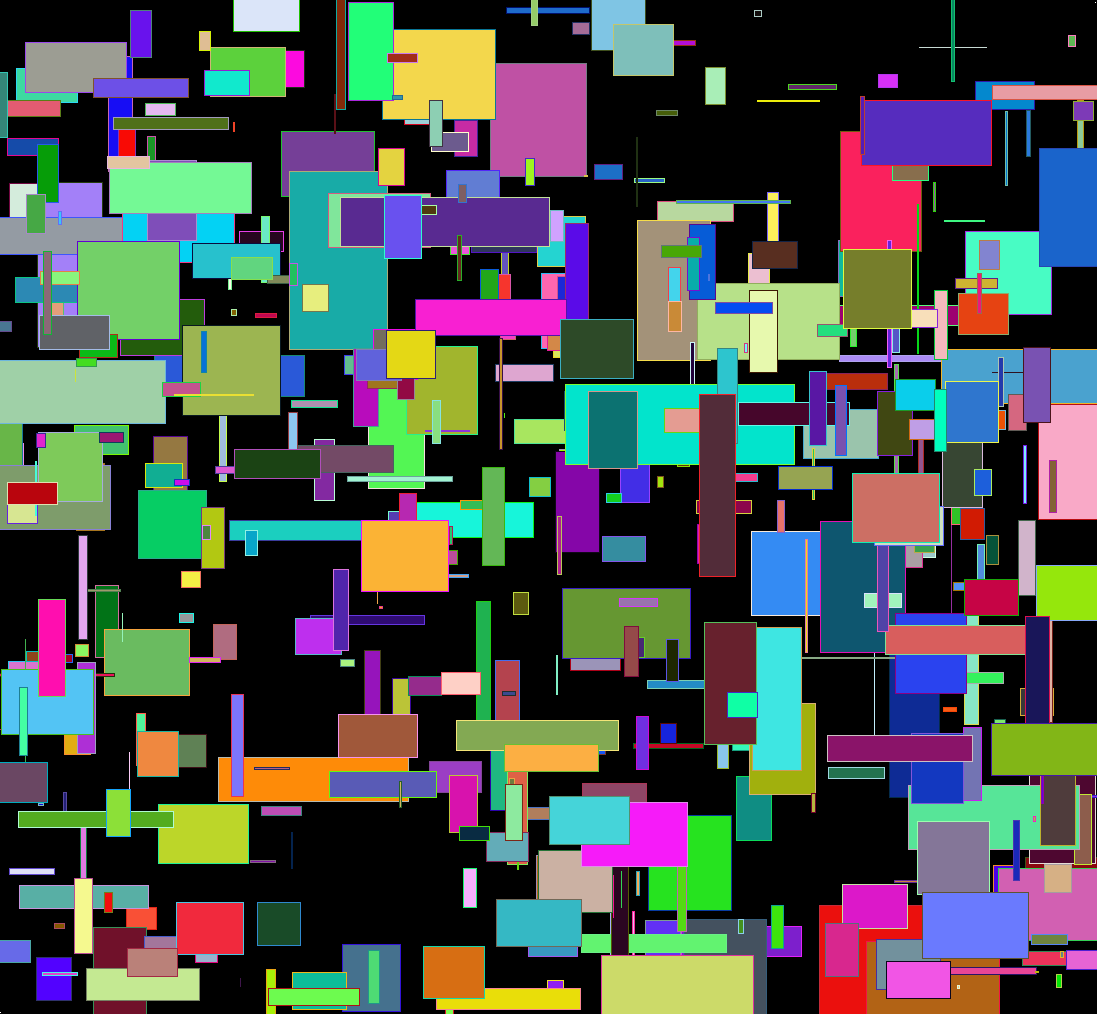[This article was first published on Probability and statistics blog » r, and kindly contributed to R-bloggers]. (You can report issue about the content on this page here)
Want to share your content on R-bloggers? click here if you have a blog, or here if you don't.As usual click on the image for a full-size version. Code:

```par(bg="black")
par(mar=c(0,0,0,0))
plot(c(0,1),c(0,1),col="white",pch=".",xlim=c(0,1),ylim=c(0,1))
iters = 500
for(i in 1:iters) {
center = runif(2)
size = rbeta(2,1,50)

# Let's create random HTML-style colors
color = sample(c(0:9,"A","B","C","D","E","F"),12,replace=T)
fill = paste("#", paste(color[1:6],collapse=""),sep="")
brdr = paste("#", paste(color[7:12],collapse=""),sep="")

rect(center-size, center-size, center+size, center+size, col=fill, border=brdr, density=NA, lwd=1.5)
}```